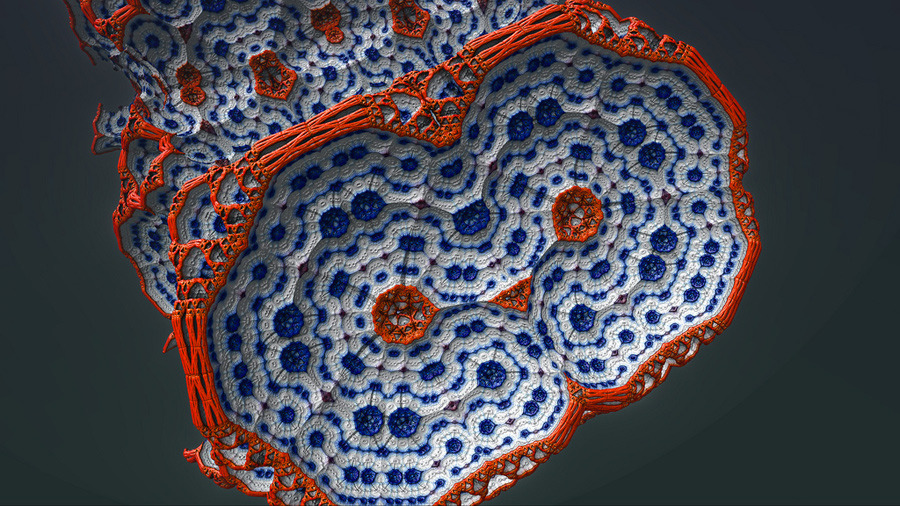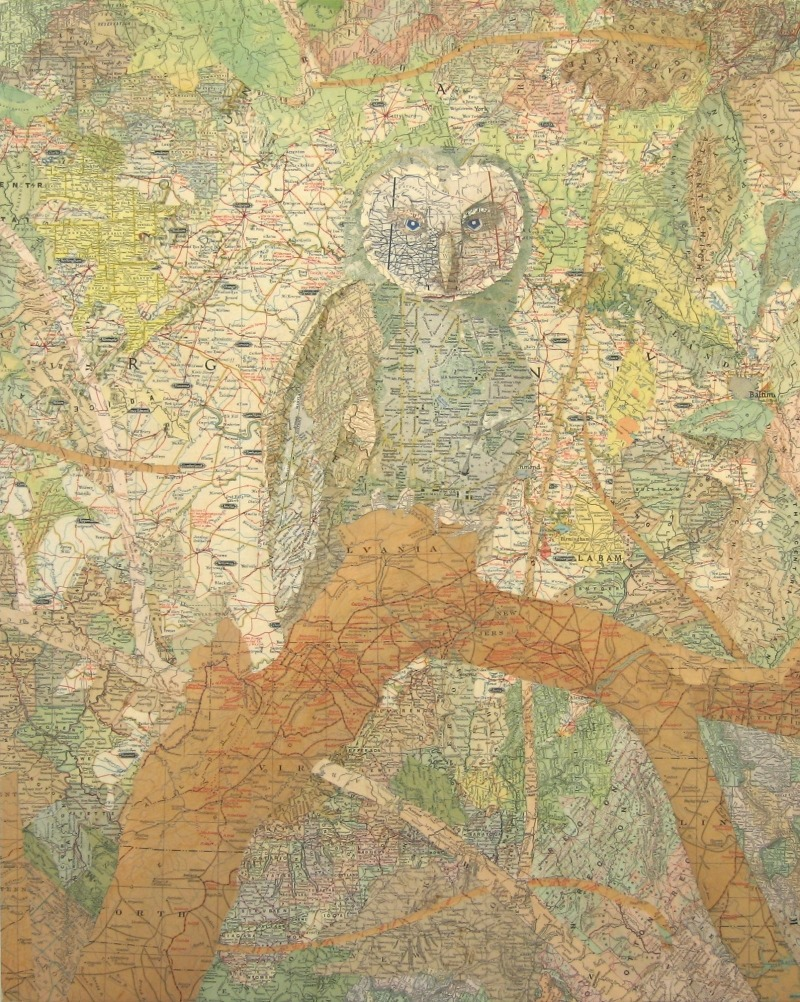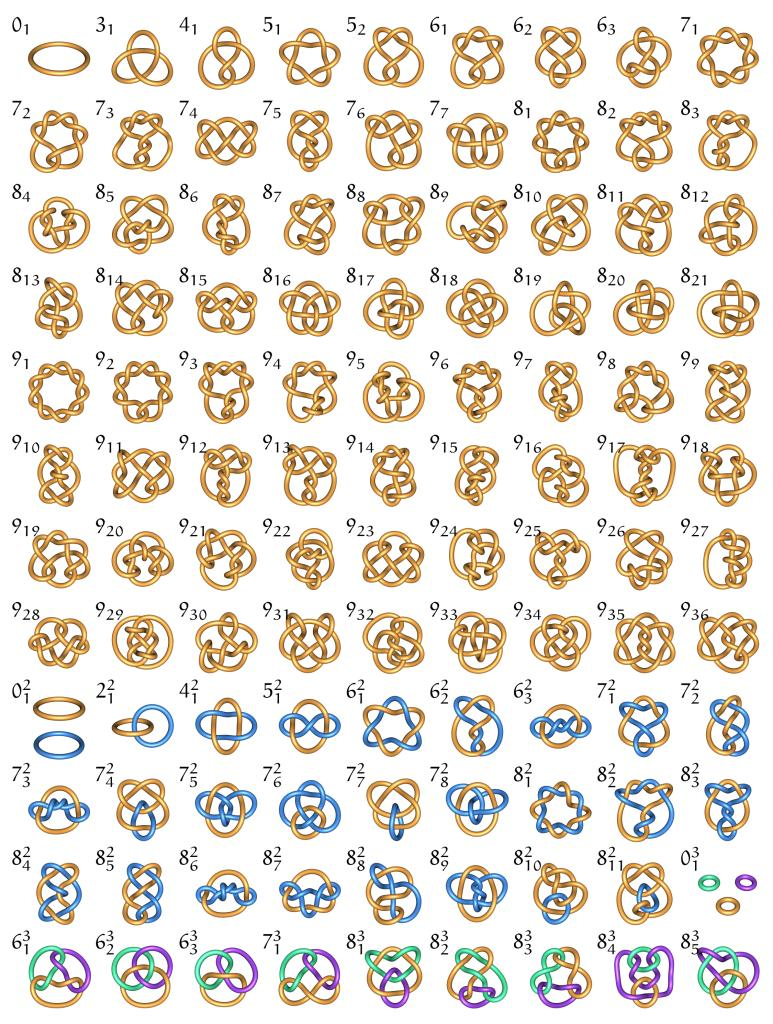## Wonder

Jun 202011via dethjunkie

## Kalmakoff

Jun 202011via butdoesitfloat

## Make Something Awesome

Jun 202011via butdoesitfloat

Make your own fractals online with Fractal Lab….

## Frac-hole

Jun 132011via senorgif

## "Fractal" Limit Orders

Jun 122011## Beautiful Fractals From Ugliano

Jun 092011via environmentalgraffiti

## Anatomia Vegetal

Jun 032011via bibliodyssey

## Amazing Map Collages

Jun 012011via butdoesitfloat

## Knot Zoo

May 312011via knotzoo

## Strangers in the Plane

Apr 232011via imgfave

Apr 112011Check out these kidz threading the E8 root system.

## Waggle Dances, Flag Manifolds, Quantum Fields

Jan 022011via ican

Dur also hooked me up with this article on Honeybees and Quantum Fields. The basic premise is that honeybee dances are 2-dimensional projections of flag manifolds. Somehow this also involves quantum fields because quarks have something to do with flag manifolds. The whole thing sounds like a stretch to me, but I really don’t know much about bees or quantum fields. On the other hand, I do love cats dancing in bee costumes.

## Proofs I: The Pythagorean Theorem

Dec 312010The Pythagorean Theorem is a true classic in the history of math. Generally, peeps credit the Ionian mathematician, Pythagoras, with the first proof of this theorem back around 500 BC. The oldest proof on wikipedia is Euclid’s proof and it looks pretty complicated. This is because Euclid was working within the classical framework of Euclidean geometry. As modern mathematicians, we have more powerful and diverse tools at our disposal for this proof. However, this makes the setup for our proof somewhat more complicated. Where do we begin? How much do we assume before beginning? I guess I’ll assume a basic knowledge of modern geometry. In particular, I’ll assume a basic knowledge of angles, lengths and triangles. The theorem concerns the length of the sides of a right triangle. Recall that the two sides connected to the right angle are called legs and the third side, opposite the right angle, is called the hypotenuse. Here’s a statement of the theorem:

Theorem: The sum of the squares of the legs of a right triangle is equal to the square of the hypotenuse.

So, according to the labels on the picture to the left, this relationship can be written as [pmath]a^2 + b^2 = c^2[/pmath]1. The easiest proof involves putting four of these right triangles together to form a square. You can do this in two ways, as demonstrated at the left. If you put them together in the top square, then you get the following proof:

Top Proof: The large square has side of length c and therefore it has area [pmath]A = c^2[/pmath]. However, we can see that this square is also composed of four triangles and a tiny square inside them. The area of each triangle is [pmath]1/2 ab[/pmath] and the area of the square is [pmath](b-a)^2[/pmath]. So, the area of the big square can also be written [pmath]4( 1/2 ab ) + (b-a)^2 [/pmath]. We can use algebra to rewrite this as [pmath]2ab + b^2 – 2ab + a^2 = b^2 + a^2[/pmath]. Since we already discovered that the area of the big square was [pmath]c^2[/pmath], we deduce that [pmath]a^2 + b^2 = c^2[/pmath]. The sum of the squares of the legs is equal to the square of the hypotenuse.

The argument for the bottom construction is similar. Both of these are found on wikipedia, along with several other proofs if you’re interested. My favorite might be the following proof by animated gif:1. I got pmath to work! And boy does it look crappy.
 © 2011 ∅ empty set Suffusion theme by Sayontan Sinha